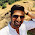VHDL coding tips and tricks: Example : 4 bit Ring Counter with testbench

## Monday, September 13, 2010

### Example : 4 bit Ring Counter with testbench

A ring counter is a digital circuit which consists of a series of flip flops connected together in a feedback manner.The circuit is special type of shift register where the output of the last flipflop is fed back to the input of first flipflop.When the circuit is reset, except one of the flipflop output,all others are made zero. For n-flipflop ring counter we have a MOD-n counter. That means the counter has n different states.
The circuit diagram for a 4 bit ring counter is shown below:

I have written a VHDL code for a 4-bit ring counter which has the following states:
0001  -   0010   -  0100   -   1000 ....
The code is posted below:
library IEEE;
use IEEE.STD_LOGIC_1164.ALL;
use IEEE.NUMERIC_STD.ALL;

entity ring_counter is
port (
DAT_O : out unsigned(3 downto 0);
RST_I : in std_logic;
CLK_I : in std_logic
);
end ring_counter;

architecture Behavioral of ring_counter is

signal temp : unsigned(3 downto 0):=(others => '0');

begin

DAT_O <= temp;

process(CLK_I)
begin
if( rising_edge(CLK_I) ) then
if (RST_I = '1') then
temp <= (0=> '1', others => '0');
else
temp(1) <= temp(0);
temp(2) <= temp(1);
temp(3) <= temp(2);
temp(0) <= temp(3);
end if;
end if;
end process;

end Behavioral;

The testbench code used for testing the design is given below:

LIBRARY ieee;
USE ieee.std_logic_1164.ALL;
USE ieee.numeric_std.ALL;

ENTITY tb IS
END tb;

ARCHITECTURE behavior OF tb IS
--Inputs
signal RST_I : std_logic := '0';
signal CLK_I : std_logic := '0';
--Outputs
signal DAT_O : unsigned(3 downto 0);
-- Clock period definitions
constant CLK_I_period : time := 1 ns;

BEGIN

-- Instantiate the Unit Under Test (UUT)
uut: entity work.ring_counter PORT MAP (
DAT_O => DAT_O,
RST_I => RST_I,
CLK_I => CLK_I
);

-- Clock process definitions
CLK_I_process :process
begin
CLK_I <= '1';
wait for CLK_I_period/2;
CLK_I <= '0';
wait for CLK_I_period/2;
end process;

-- Stimulus process
stim_proc: process
begin
RST_I <= '1';
wait for 2 ns;
RST_I <= '0';
wait for 5 ns;
RST_I <= '1';
wait for 1 ns;
RST_I <= '0';
wait;
end process;

END;

The simulation wave form is given below:

#### 2 comments:

1.Hi Vipin,
Thank you so much for this 4 bit counter coding.
Can you please help me out in coding part to count 0,1,3,4,6 and so on. Thanks in advance.

Regards,
Malarvizhi

2.I want some VHDL codes for my project Plzzz help me
1.VHDL code for Priority generator using XOR as component structural
2.VHDL code for displaying 0 to 99 nos. on LED
3.VHDL code for SIPO & PISO with circuit diagram
4.VHDL code for Carry Lookahead Adder with circuit diagram
5.VHDL code for 16:1 Mux using 4:1 & 8:1 Mux as component structural style
6.VHDL code for Universal Shift Register